1004

100

A shape with four equal sides at 90 degree angles

A square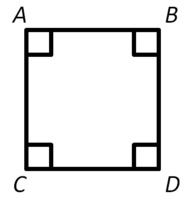100

Liam had read that oranges are good for him. He went to buy 8 oranges at the grocery store. Each orange costs 50 cents. How much did Liam have to pay?

\$ 4.00

100

What will be the FIRST THING YOU DO on the state math assessment?

Make a multiplication chart!

100

48 is 6 times the value of 8.

Write as an equation

48=6x8 or 48=8x6

or

6x8=48 or 8x6=48

200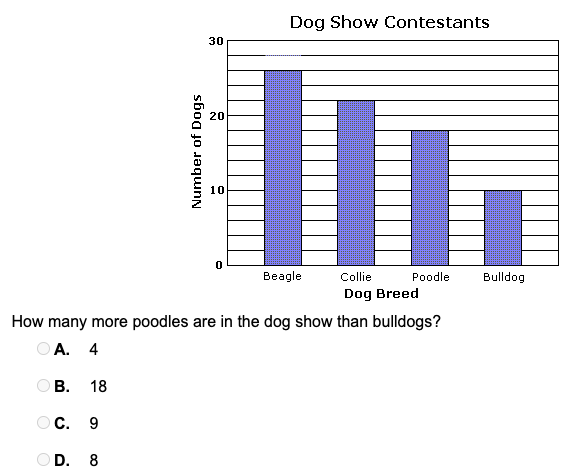8

200

The area of a square with a side length of 12 in.

144 in3

200

Liam went to the doctor. There are 8 patients waiting. The nurse told him it will be his turn after 2 hours. How many minutes will it take for the doctor to see Liam?

120 minutes

200

221 x 2 =

442

200

Sum

300

11 x 12=

132

300

The perimeter of a regular hexagon with a side length of 9 cm.

54 cm

300

Jan usually gets up at 7:30 a.m. Since she was sick, she got up 1 hour and 30 minutes later than usual. What did she get up?

9:00 am

300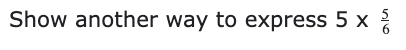25/6

25 x 1/6

5 x 5 x 1/6

300

60 minutes

What is one hour?

400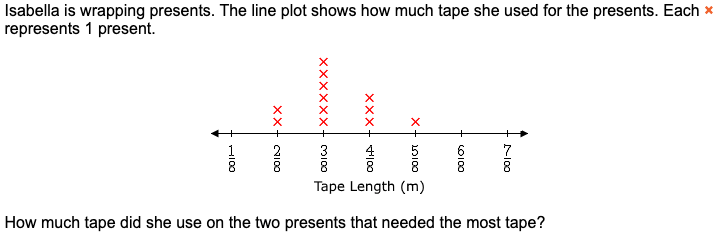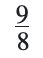400

The measurement of a straight angle.

180 degrees

4004 oranges

400
A quarter of an hour is how long?

15 minutes

400

Product

What is the answer to a multiplication problem?

500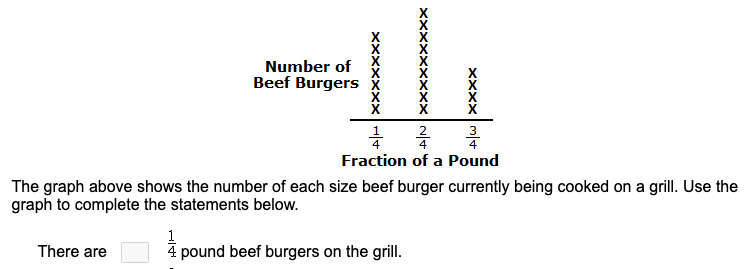7

500

A figure with a point at one end, and an arrow at the other end is called a/an.........

ray

500

Liam has some medicine at home for his headache and fever. There are 36 tablets left in the bottle and he is supposed to take 3 tablets a day. How many tablets will be left after day 11?

3 tablets

500

1001- 981=

20

500
Part of a whole
What is the definition of a fraction?
Click to zoom